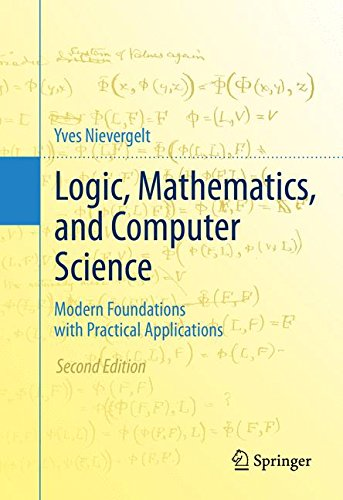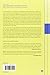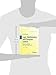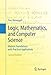Thursday , February 20th 2020

# Logic, Mathematics, and Computer Science: Modern Foundations with Practical Applications#### Product Features:

This text for the first or second year undergraduate in mathematics, logic, computer science, or social sciences, introduces the reader to logic, proofs, sets, and number theory. It also serves as an excellent independent study reference and resource for instructors. Adapted from Foundations of Logic and Mathematics: Applications to…
Price as on: 2020-01-05 15:46:31

#### Product Description

This text for the first or second year undergraduate in mathematics, logic, computer science, or social sciences, introduces the reader to logic, proofs, sets, and number theory. It also serves as an excellent independent study reference and resource for instructors. Adapted from Foundations of Logic and Mathematics: Applications to Science and Cryptography © 2002 Birkhӓuser, this second edition provides a modern introduction to the foundations of logic, mathematics, and computers science, developing the theory that demonstrates construction of all mathematics and theoretical computer science from logic and set theory.  The focuses is on foundations, with specific statements of all the associated axioms and rules of logic and set theory, and  provides complete details and derivations of formal proofs. Copious references to literature that document historical development is also provided.

Answers are found to many questions that usually remain unanswered: Why is the truth table for logical implication so unintuitive? Why are there no recipes to design proofs? Where do these numerous mathematical rules come from? What issues in logic, mathematics, and computer science still remain unresolved? And the perennial question: In what ways are we going to use this material?   Additionally, the selection of topics presented reflects many major accomplishments from the twentieth century and includes applications in game theory and Nash’s

equilibrium, Gale and Shapley’s match making algorithms, Arrow’s Impossibility Theorem in voting, to name a few.

From the reviews of the first edition:

“…All the results are proved in full detail from first principles…remarkably, the arithmetic laws on the rational numbers are proved, step after step, starting from the very definitions!…This is a valuable reference text and a useful companion for anybody wondering how basic mathematical concepts can be rigorously developed within set theory.”

MATHEMATICAL REVIEWS

“Rigorous and modern in its theoretical aspect, attractive as a detective novel in its applied aspects, this paper book deserves the attention of both beginners and advanced students in mathematics, logic and computer sciences as well as in social sciences.”

Zentralblatt MATH

## The Design and Analysis of Computer

With this text, you gain an understanding of the fundamental concepts of algorithms, the very heart of computer science.…

## Mathematics for 3D Game Programming and Computer

This updated third edition illustrates the mathematical concepts that a game developer needs to develop 3D computer graphics and…